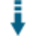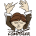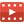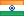# PHP Breadth First Search: Find the shortest path to visit warehouse binsDownload .zip
 InfoExampleScreenshotsView files (9)Download .zip Reputation Support forum Blog Links
 Ratings Unique User Downloads Download Rankings Not yet rated by the users Total: 31 All time: 10,930 This week: 80Description

Author

This package can find the shortest path to visit warehouse bins.

It can generate a graph with a given number of nodes and a list of edges that can have bins in a warehouse.

The package can find the shortest path to traverse all bins from a starting point and return to the same point.

A Python component can render the warehouse bins' path as an image.Performance Level
Name: Classes: Malik Naik is available for providing paid consulting. Contact Malik Naik . 9 packages by Malik NaikIndia 25 3541 232 in India65851 in IndiaInnovation awardNominee: 5x

## Example

 ``` addEdge ( \$edge, \$edge ); } // Get all the bins. \$bins = array_keys( \$bin_mapping ); \$bins = array_diff( \$bins, ['start'] ); // Initialize the Sylvance class object. \$sylvane = new Sylvane( \$bins ); // Get 12 random bins. \$random_bins = \$sylvane->getRandomBins ( 12 ); // Instantiate the PathFinder class object. \$path_finder = new PathFinder ( \$graph ); // Get all distances. \$distances = \$path_finder->getDistances(); // Get all paths. \$paths = \$path_finder->getPaths(); // Initally no bin is visited. \$visited = []; // Start from the start position in the warehouse. \$current_node = 'start'; // Store the final path. \$final_path = ['start']; // Store the path in the graph with node ids. \$exact_path = ; while(count(\$visited) <= 12) {     if(count(\$visited) < 12) {         // Get the nearest bin.         \$nearest = getNearestBin(\$current_node, \$random_bins, \$visited, \$distances, \$bin_mapping);     } else {         \$nearest = 'start';     }     // Mark the nearest bin as visited.     \$visited[] = \$nearest;     // Get the path from the current node to the nearest bin.     \$current_path = \$paths[\$bin_mapping[\$current_node]][\$bin_mapping[\$nearest]];     // Add the path to the exact path.     for(\$i = 1; \$i < count(\$current_path); \$i++) {         \$exact_path[] = \$current_path[\$i];     }     // Update the current node.     \$current_node = \$nearest;     // Add the nearest bin to the final path.     \$final_path[] = \$nearest; } echo "Random Bins selected are:\n---\n"; foreach(\$random_bins as \$bin) {     echo \$bin . "\n"; } echo "\n\nThe optimal bin visiting order is as follows:\n---\n"; \$i = 0; foreach(\$final_path as \$bin) {     echo \$bin;     if(\$i == count(\$final_path) - 1) {         echo "\n";     } else {         echo " -> ";     }     \$i++; } echo "\n\nThe exact path in the graph is as follows with a total distance of " . count(\$exact_path) . " steps:\n---\n"; \$i = 0; foreach(\$exact_path as \$node) {     echo \$node;     if(\$i == count(\$exact_path) - 1) {         echo "\n";     } else {         echo " -> ";     }     \$i++; } // Random Bins selected are: // --- // B1 // B4 // A6 // A7 // A10 // C1 // C5 // C11 // F2 // F9 // E11 // G5 // The optimal bin visiting order is as follows: // --- // start -> C1 -> C5 -> C11 -> E11 -> F9 -> F2 -> B1 -> B4 -> A6 -> A7 -> A10 -> G5 -> start // The exact path in the graph is as follows with a total distance of 73 steps: // --- // 14 -> 15 -> 16 -> 17 -> 18 -> 19 -> 20 -> 21 -> 22 -> 23 -> 24 -> 25 -> 60 -> 61 -> 38 -> 37 -> 36 -> 35 -> 34 -> 33 -> 32 -> 31 -> 30 -> 29 -> 28 -> 27 -> 26 -> 55 -> 54 -> 13 -> 53 -> 52 -> 0 -> 1 -> 2 -> 3 -> 4 -> 5 -> 6 -> 7 -> 8 -> 9 -> 10 -> 11 -> 12 -> 58 -> 59 -> 25 -> 60 -> 61 -> 38 -> 62 -> 63 -> 51 -> 50 -> 49 -> 48 -> 47 -> 46 -> 45 -> 44 -> 43 -> 42 -> 41 -> 40 -> 39 -> 57 -> 56 -> 26 -> 55 -> 54 -> 13 -> 14 ```

## Details

This is a task to find the shortest path by visiting all the bins in the warehouse and coming back to the start position in an optimal way.

## Approach

1. We are considering the aisle as the nodes in the graph and when we are at a certain aisle we can pick the item from the bin on either side of the aisle.
2. The Modified Breadth-First Search is used to find the shortest path from all pairs of nodes in the graph.
3. Starting with the start position this approach greedily picks the nearst node from the start position and then takes the path and then from there picks the next nearsest node until all the bins are visited.
4. Finally, it returns back to the start position.

The graph of the warehouse is as follows:In the above graph, the green nodes are the aisles and are paths that can be traversed and the white nodes are the bins from which we can pick the items.

## Execution

To test the working of this code. Clone this repository and then run the following command:

``````php main.php
``````

Make sure you have PHP CLI installed before running the above command.

## Visualization

To execute the visualization using Python you need to install the `NetworkX` and `Matplotlib` libraries. They can be installed by running the following command:

``````python -m pip install -r requirements.txt
``````

Then, run the following command to generate the visualization of the Warehouse.

``````python visualization.py
``````Files
File Role Descriptionbins.py Data Auxiliary dataGraph.php Class Class sourcemain.php Example Example scriptparams.php Aux. Auxiliary scriptPathFinder.php Class Class sourceREADME.md Doc. Documentationrequirements.txt Doc. DocumentationSylvane.php Class Class sourcevisualization.py Data Auxiliary data

 All time: 10,930 This week: 80About usAdvertise on this siteSite mapNewsletterStatisticsSite tipsPrivacy policyContact
For more information send a message to `info at phpclasses dot org`.